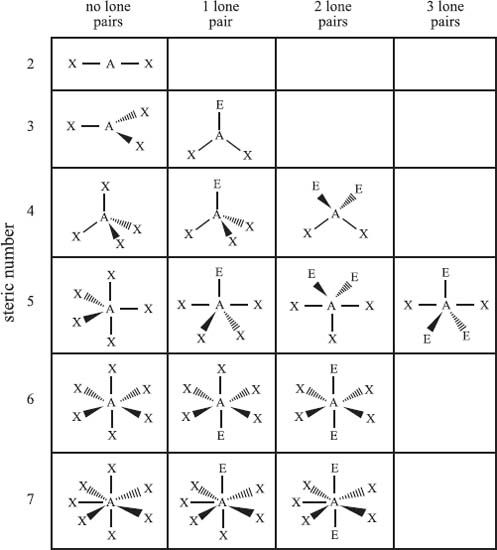# What is the valence shell electron pair repulsion model?

The valence shell electron pair repulsion model (VSEPR) is used to predict the bonding geometry about a central atom based on a set of rules that predicts the repulsive forces between the electrons in chemical bonds and in lone pairs. In this model, two factors are used to predict the bonding geometry: the steric number and the number of lone pairs of nonbonding electrons. The steric number is defined as the number of atoms bonded to the central atom plus the number of lone pairs of nonbonding electrons. Based on these two numbers, the table below predicts the bonding geometry that will be observed about a central atom. It should be noted that this is just a predictive model, and it will not be correct 100% of the time.From top row down, and left to right, the types of bonding geometries are: Steric Number 2) linear; Steric Number 3) trigonal planar, bent; Steric Number 4) tetrahedral, trigonal pyramidal, bent; Steric Number 5) trigonal bipyramidal, seesaw, T-shaped, linear; Steric Number 6) octahedral, square pyramidal, square planar; Steric Number 7) pentagonal bipyramidal, pentagonal pyramidal, and pentagonal planar.

Close

This is a web preview of the "The Handy Chemistry Answer Book" app. Many features only work on your mobile device. If you like what you see, we hope you will consider buying. Get the App